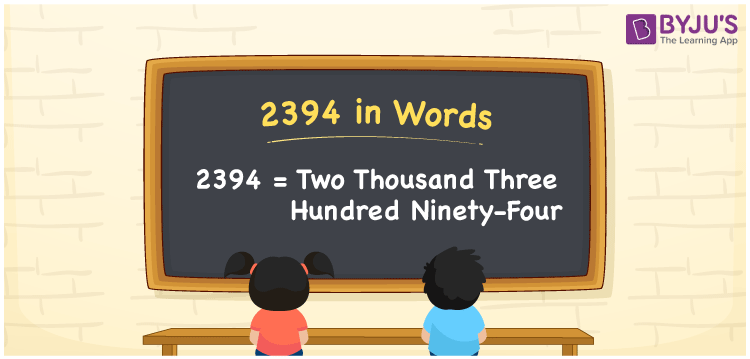# 2394 in Words

2394 in words is Two thousand three hundred ninety-four. As we all know, the number 2394 is a cardinal number. For example, the cost of shirts purchased is Rs. 2394. It can also be written as “The cost of shirts purchased is Rs. Two thousand three hundred ninety-four. Now, let us have a look at the process of writing the number 2394 in words using the place value system.

 2394 in Words: Two Thousand Three Hundred Ninety-four. Two Thousand Three Hundred Ninety-four in Numerical Form: 2394.

## 2394 in English Words## How to Write 2394 in Words?

Following is the place value table for the number 2394:

 Thousands Hundreds Tens Ones 2 3 9 4

The expanded form of 2394 is as follows:

= 2 × Thousand + 3 × Hundred + 9 × Ten + 4 × One

= 2 × 1000 + 3 × 100 + 9 × 10 + 4 × 1

= 2000 + 300 + 90 + 4

= 2394

= Two thousand three hundred ninety-four

Hence, 2394 in words is two thousand three hundred ninety-four.

After 2393 but before 2395, the number 2394 occurs.

2394 in words – Two thousand three hundred ninety-four

Is 2394 an odd number? – No

Is 2394 an even number? – Yes

Is 2394 a perfect square number? – No

Is 2394 a perfect cube number? – No

Is 2394 a prime number? – No

Is 2394 a composite number? – Yes

## Frequently Asked Questions on 2394 in Words

### Write 2394 in words.

2394 in words is two thousand three hundred ninety-four.

### Simplify 2000 + 394, and express it in words.

Simplifying 2000 + 394, we get 2394. Hence, 2394 in words is two thousand three hundred ninety-four.

### Is 2394 an even number?

Yes, 2394 is an even number.